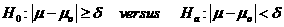# Sample Size Calculator: One Sample Mean

Hypothesis: One-Sided EquivalenceData Input: (Help) (Example)

 Input Results α β Allowable difference N Population variance δ

Note:

 Variables Descriptions α One-sided significance level 1-β Power of the test Allowable difference Acceptable difference between sample mean and known or population mean (µ-µ0) Population variance Population varianceδ Equivalence limit N Sample size

Help Aids Top

Application: The test drug is determined to be equivalent to a gold standard on average if the null hypothesis is rejected at significance level α. The following hypotheses are usually considered:Procedure:

1. Enter

a)      value of α, the probability of type I error

b)      value of β, the probability of type II error

c)      value of allowable difference

d)     value of Population variance

e)      value of δ>0, the equivalence limit.

1. Click the button “Calculate” to obtain result sample size N.

Formula:(*)

Notations:

α:               The probability of type I error (significance level) is the probability of rejecting the true null hypothesis.

β:               The probability of type II error (1 – power of the test) is the probability of failing to reject the false null hypothesis.

δ:               The largest change from the reference value (baseline) that is considered to be trivial.

μ – μ0:       The value of allowable difference is the difference value between true mean and reference mean (constant value).

Examples

Example 1:  Concerning the effect of a test drug on body weight change in terms of body mass index (BMI) before and after the treatment, we consider that a less than 5% change in BMI from pre-treatment is not safety concern for the indication of the disease under study. Thus, δ=5% as the equivalence limit and to demonstrate safety by testing equivalence in mean BMI between pre-treatment and post-treatment of the test drug. Now, if true BMI difference is 0 (μ–μ0=0) and the population variance is 0.01, by (*) with α=0.05, we required the sample size of N=35 to achieve an 80% power (β=0.2).

Reference: Chow, Shao and Wang, Sample Size Calculations In Clinical Research, Taylor & Francis, NY. (2003) Pages 52-53.